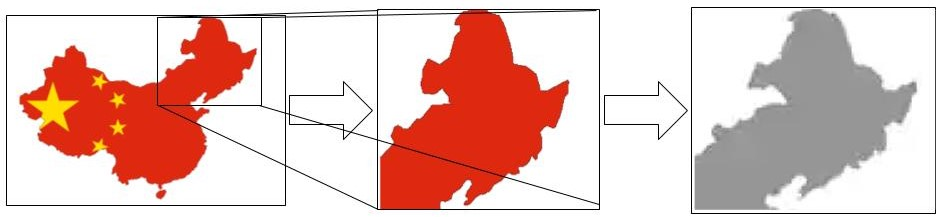20 / 32

# Numpy - Arrays - Example - Reshaping a complex array

Now, as we just finished learning some simple examples of using numpy array's `reshape()` function, let us now learn a more complex use of `reshape()` function.

For this, we will load a colored image, convert it into a grayscale image, and then will apply to `reshape()` function on this grayscale image.INSTRUCTIONS

(1) Please import the required libraries

``````import numpy as np
``````

(2) Let us use the `load_sample_images` function to load `china.jpg` image and store the loaded image in a variable called `china`

``````china = load_sample_image("china.jpg")
``````

(3) Please check the shape of `china` array

``````china.<<your code comes here>>
``````

the shape of `china` array is `(427, 640, 3)`

Let us see the structure of this `china` array and then we will try to understand each value in this shape.

`china` is a 3-dimensional numpy array, and it looks something like below

``````[
[ [174, 201, 231], [250, 251, 255],...........]    - row 0
[ [172, 199, 229], [251, 252, 255],...........]    - row 1
.
.
]
``````

Hence, in `china` array, we see that

• there are some rows - the second level brackets ('`[`') represents rows. Like this, we have 427 rows.
• each row has some pixels (each sub-array like `[174, 201, 231]` in the row represents a pixel). Like this we have 640 pixels in each row.
• the 3 values inside each pixel array ( [`174, 201, 231`] ) represent colour codes for the colour of this pixel. e.g. 174, 201 and 231 combinedly may be representing a dark brown colour (colour of this pixel of the china image).

If you see the values above (marked in bold font), you will know what each value of the shape (427, 640, 3) represents in the china array.

(4) Just for simplifying the things, let us extract a small portion of this `china` numpy array and store it in a variable called `image`. Here, we are extracting (slicing) a portion (`image`) of `china` array from row number 150 to 220 and column number 130 to 250.

``````image = china[150:220, 130:250]
``````

Now, let us check the shape of this `image` array by using the below code

``````image.<<your code comes here>>
``````

(5) Please store each value of shape 'tuple' separately in variables - `height`, `width` and `channels`

``````height, width, channels = image.shape
``````

Here, `channels` are nothing but the number of colour codes i.e. 3.

(6) Let us convert the coloured `image` into a grayscale image (`image_grayscale`), by replacing the values which are along the `axis=2`, by their mean value.

Do you remember the concept of axis explained earlier?

• `axis=0` represents the first dimension of an array, i.e. it represents the row
• `axis=1` represents the second dimension of an array, i.e. it represents the column
• `axis=2` represents the third dimension of an array, in this case, the third dimension is `channels`.

`channels` (`axis=2`) has 3 values (e.g. [68, 39, 44] ), and, mean of these 3 values is 50.33 Hence, value [68, 39, 44] will be replaced with value 50.33 after the step - `image.mean(axis=2)`

``````   image_grayscale = image.mean(axis=2).astype(np.float32)
``````

Now, let us check the shape of this `image_grayscale` array by using the below code

``````image_grayscale.<<your code comes here>>
``````

(7) Now, let us reshape this `image_grayscale` array into a 4-dimensional array (from existing 2-dimensions) and store the output in a variable called `images`.

``````<<your code comes here>> = image_grayscale.reshape(1, height, width, 1)
``````

(8) Please check dimensions of `images` array

``````images.<<your code comes here>>
``````

No hints are availble for this assesment

Note - Having trouble with the assessment engine? Follow the steps listed here